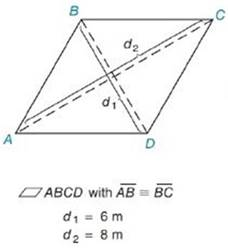Chapter 8.2, Problem 3EElementary Geometry For College St...

7th Edition
Alexander + 2 others
ISBN: 9781337614085

Solutions

Chapter
SectionElementary Geometry For College St...

7th Edition
Alexander + 2 others
ISBN: 9781337614085
Textbook Problem

In Exercises 1 to 8, find the perimeter of each polygon.To determine

To Find:

Perimeter of the rhombus provided.

Explanation

Formula Used:

The perimeter of a polygon is the sum of the lengths of all sides of the polygon.

The area A of a rhombus whose diagonals has lengths d1 and d2 is given by A=12d1d2.

The area A of a rhombus whose sides 'a' are known is given by A=a2.

Calculation:

It is given that AB-=BC-, d1=6 m and d2=8 m.

As AB-=BC- and it is given as a parallelogram, all sides must be in equal length

Still sussing out bartleby?

Check out a sample textbook solution.

See a sample solution

The Solution to Your Study Problems

Bartleby provides explanations to thousands of textbook problems written by our experts, many with advanced degrees!

Get Started

Change 115 mi/s to mi/min.

Elementary Technical Mathematics

In Exercises 1728, use the logarithm identities to obtain the missing quantity.

Finite Mathematics and Applied Calculus (MindTap Course List)

The general solution to (for x, y > 0) is: a) y = ln x + C b) c) y = ln(ln x + C) d)

Study Guide for Stewart's Single Variable Calculus: Early Transcendentals, 8th